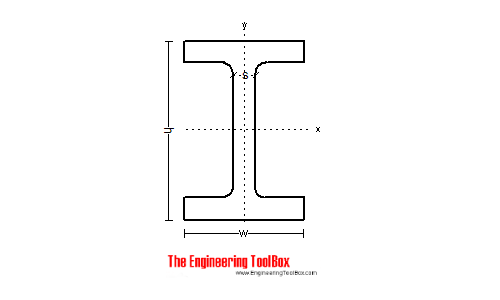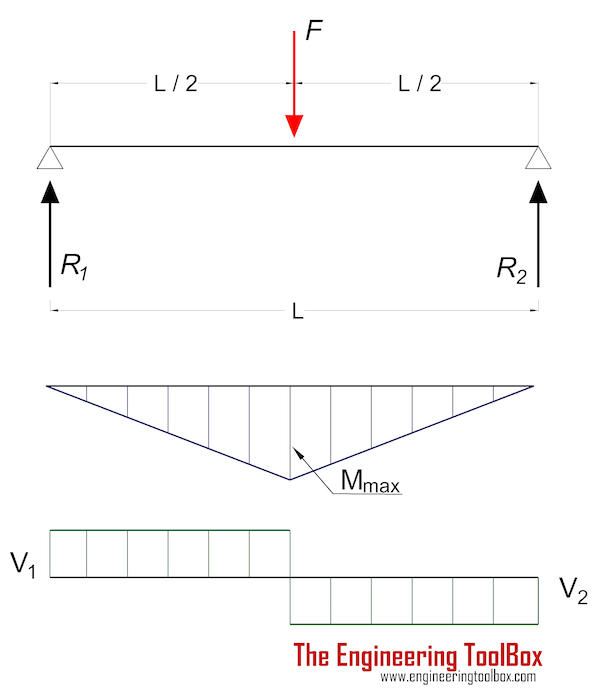Engineering ToolBox - Resources, Tools and Basic Information for Engineering and Design of Technical Applications!

# Stress

## Stress is force applied on cross-sectional area.

Stress is the ratio between applied force and cross-sectional area where the applied force is acting.

### Normal Stress

Normal stress can be expressed as

σ = FN / A                           (1)

where

σ = normal stress (N/m2, Pa, psi)

FN = applied force perpendicular to the area - Normal force (N, lb)

A = cross-sectional area (m2, in2)

### Shear Stress

Shear stress can be expressed as

τ = FV / A                           (2)

where

τ = shear stress (N/m2, Pa, psi)

FV = applied force in plane of the area - Shear force (N, lb)

### Example - Normal Stress in a ColumnA 10000 N force is acting in the direction of a British Universal Column UB 152 x 89 x 16 with cross sectional area 20.3 cm2. The normal stress in the column can be calculated as

σ = (10000 N) / ((20.3 cm2) (0.0001 m2/cm2

= 4926108 Pa (N/m2)

= 4.9 MPa

The Yield strength - the amount of stress that a material can undergo before moving from elastic deformation into plastic deformation - is typical 250 MPa for steel.

### Example - Shear Stress in a Beam with Point LoadFor a beam with single point load supported on both ends - the shear force Fv (or V in the figure above) is equal in magnitude to support force R1 or R2.

Reaction forces can be calculated due to moment equilibrium around support 1

F L / 2 = R2 L                              (4)

R2 = F / 2                              (5)

R1 = R2 = F / 2                         (6)

For a 10000 N point load perpendicular on a beam similar to the example above - supported at both ends - the magnitude of the reaction and shear forces can be calculated as

R1 = R2

= V1

= V2

= (10000 N) / 2

= 5000 N

= 5 kN

The shear stress can be calculated as

τ = (5000 N) / ((20.3 cm2) (0.0001 m2/cm2)

= 2463054 Pa

= 2.5 MPa

## Related Topics

• Mechanics - Forces, acceleration, displacement, vectors, motion, momentum, energy of objects and more.
• Statics - Loads - forces and torque, beams and columns.

## Engineering ToolBox - SketchUp Extension - Online 3D modeling!

Add standard and customized parametric components - like flange beams, lumbers, piping, stairs and more - to your Sketchup model with the Engineering ToolBox - SketchUp Extension - enabled for use with the amazing, fun and free SketchUp Make and SketchUp Pro .Add the Engineering ToolBox extension to your SketchUp from the SketchUp Pro Sketchup Extension Warehouse!

Translate

## Privacy

We don't collect information from our users. Only emails and answers are saved in our archive. Cookies are only used in the browser to improve user experience.

Some of our calculators and applications let you save application data to your local computer. These applications will - due to browser restrictions - send data between your browser and our server. We don't save this data.

## Citation

• Engineering ToolBox, (2008). Stress. [online] Available at: https://www.engineeringtoolbox.com/stress-d_1395.html [Accessed Day Mo. Year].

Modify access date.

. .

#### Scientific Online Calculator3 30